# Sources

Time-dependent and controlled voltage and current sources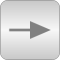# Package Contents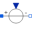SignalVoltage Generic voltage source using the input signal as source voltage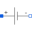ConstantVoltage Source for constant voltage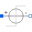StepVoltage Step voltage source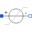RampVoltage Ramp voltage source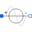SineVoltage Sine voltage source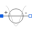CosineVoltage Cosine voltage source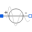ExpSineVoltage Exponentially damped sine voltage source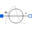ExponentialsVoltage Rising and falling exponential voltage source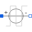PulseVoltage Pulse voltage source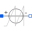SawToothVoltage Saw tooth voltage source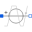TrapezoidVoltage Trapezoidal voltage source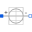TableVoltage Voltage source by linear interpolation in a table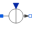SignalCurrent Generic current source using the input signal as source current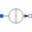ConstantCurrent Source for constant current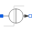StepCurrent Step current source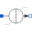RampCurrent Ramp current source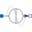SineCurrent Sine current source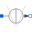CosineCurrent Cosine current source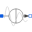ExpSineCurrent Exponentially damped sine current source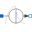ExponentialsCurrent Rising and falling exponential current source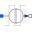PulseCurrent Pulse current source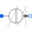SawToothCurrent Saw tooth current source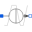TrapezoidCurrent Trapezoidal current source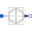TableCurrent Current source by linear interpolation in a table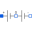SupplyVoltage Supply voltage (positive and negative)

# Information

This information is part of the Modelica Standard Library maintained by the Modelica Association.

This package contains time-dependent and controlled voltage and current sources. Most of the sources use the behavior modeled in the Modelica.Blocks.Sources package. All sources are ideal in the sense that no internal resistances are included.